## Why doesn't break work?...

The background of my question comes from graph theory. But the essence of the problem has nothing to do with graph theory, and it only involves programming.

For the graph g1 below, the paired crossing edges  are marked as wine red.

with(GraphTheory):
with(SpecialGraphs):
g:=CompleteGraph(6);
g1:=DeleteEdge(g, {3,2}, inplace = false);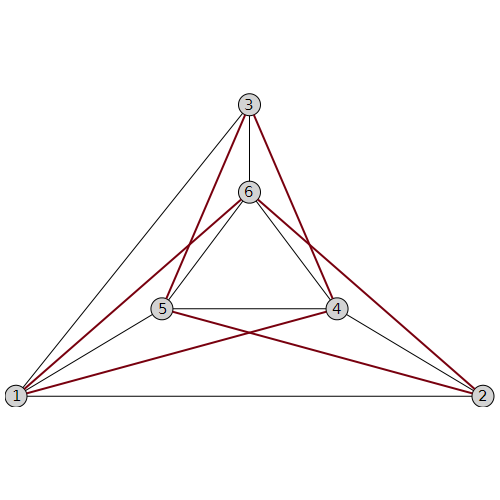Paircrossedges:=[[{1,6},{3,5}],[{2,5},{1,4}],[{2,6},{3,4}]];

For each pair of crossed edges, I choose one to delete and get a plane graph g1', and then I consider the edge connectivity of g1', if the edge connectivity is equal 3, stop the calculation and return to the deleted edge set, otherwise continue to look for.

The following code is my attempt, break does not seem to work.

Conremovedges:= proc(G::Graph,Paircrossedges)
local i,j,k,dedges;
for i from 1 to 2 do
for j from 1 to 2 do
for k from 1 to 2 do
dedges:={Paircrossedges[i],Paircrossedges[j],Paircrossedges[k]};
if EdgeConnectivity(DeleteEdge(G, dedges, inplace = false))=3 then
print(dedges);
break;
end if;
end do:
end do:
end do:
end proc:
Conremovedges(g1,Paircrossedges)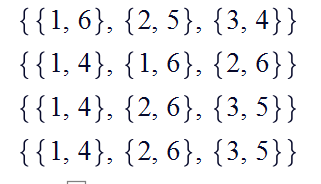My idea is that as long as the edge set that meets the condition appears for the first time, it should stop. If none are satisfied, return "not found".

Another question：

The above is just my example. In fact, the graph I want to consider is more complicated. If there are 18 pairs of crossing edges, do I need to write 18th-order for-loops? This seems very troublesome. Is there an easier way? Maximum number of for loops is 2^18 that is equal to 262144, which is still acceptable.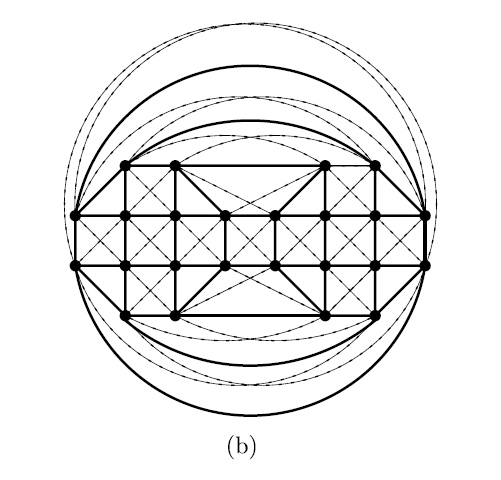Conremovedges:= proc(G::Graph,Paircrossedges)
local a1,a2,a3,a4,a5,a6,a7,a8,a9,a10,a11,a12,a13,a14,a15,a16,a17,a18,dedges;

for a1 from 1 to 2 do
for a2 from 1 to 2 do
for a3 from 1 to 2 do
for a4 from 1 to 2 do
for a5 from 1 to 2 do
for a6 from 1 to 2 do
for a7 from 1 to 2 do
for a8 from 1 to 2 do

for a9 from 1 to 2 do
for a10 from 1 to 2 do
for a11 from 1 to 2 do
for a12 from 1 to 2 do
for a13 from 1 to 2 do
for a14 from 1 to 2 do
for a15 from 1 to 2 do
for a16 from 1 to 2 do
for a17 from 1 to 2 do
for a18 from 1 to 2 do

dedges:={
Paircrossedges[a1],Paircrossedges[a2],Paircrossedges[a3],
Paircrossedges[a4],Paircrossedges[a5],Paircrossedges[a6],
Paircrossedges[a7],Paircrossedges[a8],Paircrossedges[a9],
Paircrossedges[a10],Paircrossedges[a11],Paircrossedges[a12],
Paircrossedges[a13],Paircrossedges[a14],Paircrossedges[a15],
Paircrossedges[a16],Paircrossedges[a17],Paircrossedges[a18]};
if EdgeConnectivity(DeleteEdge(G, dedges, inplace = false))=3 then
print(dedges);
break;
end if;
end do:
end do:
end do:
end do:
end do:
end do:
end do:
end do:
end do:
end do:
end do:
end do:
end do:
end do:
end do:
end do:
end do:
end do:
end proc:

## is there an option in Maple to obtain same order o...

In Maple, when calling LinearAlgebra:-Eigenvectors the order can change randomally each time. Ofcourse the result is always correct, but can show up in different order.

I find this confusing, as I am looking at the screen, and see order changed each time. This is the order of how Maple shows the eigenvalues and corresponding eigenvectors.

Why does Maple do this? Is there a way to have same order given each time? Here is an example. If you run this code twice, most likely you will get different order each time. If not, try the command one more time.

```restart;
Sy:=1/sqrt(2)*Matrix([[0,-I,0],[I,0,-I],[0,I,0]]);
lambda, v :=LinearAlgebra:-Eigenvectors(Sy);
```

screen shot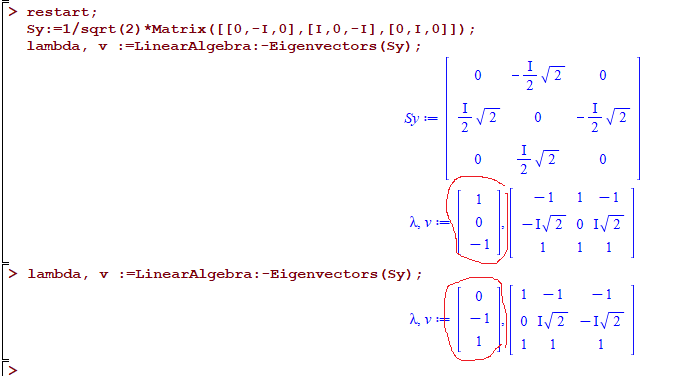I thought there might be a global option to tell Maple not to do this?

Again, answer is correct each time. I just like same output to show each time, as it is less confusing. Sometimes I think I did something wrong when I see different output show up for same command.

## how to make Maple write sqrt(2)/2 as 1/sqrt(2)? ...

sometimes I get expressions with sqrt(n)/n in them where n is positive integer. I'd like Maple to simplify this to 1/sqrt(n).

So sqrt(2)/2 will becomes 1/sqrt(2). I find this simpler.

Here is an example

```v:=Vector([1/2, -1/2*sqrt(2), 1/2]);
simplify(v);
simplify(size);
simplify(v,sqrt);
simplify(v,power);
```

and tried few other things.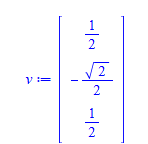is there a trick to do this? This is the case where all the expression have actual numerical integers in them (not symbolic), just like the above example. These come from numerical examples.

## Should I expect LinearAlgebra:-Equal to be more ro...

Before I put in a change request to make LinearAlgebra:-Equal the "is" of matrix/vector options, I wonder is there something else I should be doing.

LinearAlgebra_Equal.mw

## question on OOP in Maple 2021...

Few months ago, someone was kind enough to send me an email letting me know that in Maple 2021 the following new change will be in Object

```I am attending a workshop on OOP.  In Maple 2021, one will be able
to invoke a method of an instance of an object with the  syntax:  object_name:-method_name```

However, I am not able to get the above to work in Maple 2021. And I looked at the help pages and see no such example. This is what I tried

```restart;
module person()
option object;
local name := "me";

export get_name::static := proc( self::person, \$ )
self:-name;
end;

export set_name::static := proc( self::person, name, \$ )
self:-name:=name;
end;

end:

p:=Object( person ); #create object

p:-get_name();
```

Error, invalid input: person:-get_name uses a 1st argument, self (of type person), which is missing

What changes are needed in the above code to be able to use  obj:-method()  syntax?

Or is it possible that this change did not go into Maple 2021?

## Drawing toolbar missing Maple 2021...

Hey

I can't find the toolbar for drawing i Maple 2021.

Can anyone help me? I'm using Macbook.

I try to clik on at picture but I can't see it.

I try to insert a drawnig but I can't see it.

Hope someone can help me.

## Maple 2021 Software Change Requests...

Would be nice if someone could add Maple 2021 to Software Change Requests list.

Right now Maple 2021 is unusable for us due to a big problem related to saving workbooks.

## Maple2021 and CUDA...Examples

 >>>>(1.1)
 >(1.2)
 >(1.3)
 >(1.4)
 >(1.5)
 >(1.6)
 >(1.7)
 >(1.8)Hello all :-)

I just upgraded to 2021 and ran the example help page for CUDA.

From the help we can see that the ratio is 11.

With Maple 2020 I had a ratio of 7.24

With Maple 2021 I have a ratio of around 4. (see the mw attached).

I wonder with which version the help page was made but on my machines the ratio diminishes from version to version

Now it's Windows 10 Pro 20H2, the amazing AMD Ryzen Threadripper (64 cores) and 256 Go RAM, the graphic card is a NVIDIA RTX 3090 with...10496 CUDA cores.

So what can't I get a better ratio with/without CUDA?

Regards.

## Can we create more graphs with this function Sequ...

I get the degree sequence of a graph, I want to create more graphs, but maple seems to only get the only graph.

Is there a good way to get more, a foolish dream, can we get all the graphs that satisfy this degree sequence condition?

with(GraphTheory):
L:=[7\$1..22,6]:
IsGraphicSequence(L):
G := SequenceGraph(L):
DrawGraph(%)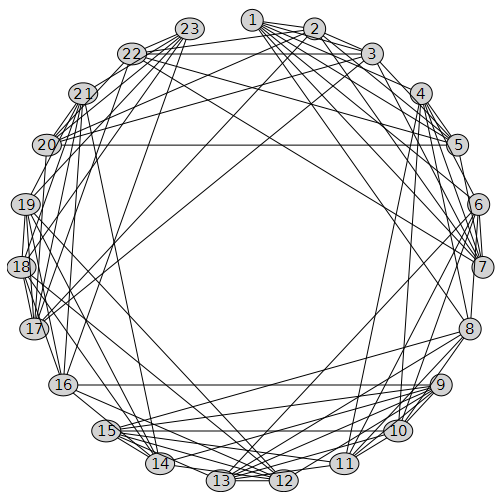## Problem with animation toolbar in Maple 2021...

Anyone have a problem with non-appearance of the animation toolbar in Maple 2021????

Whilst coming up with a response for the problem here

https://www.mapleprimes.com/questions/231862-Have-You-Ever-Heard-Of-Vector-Asterisks-

I found an issue with the non-appearance of the animation toolbar in Maple 2021. This is rather difficult to illustrate without the use of screenshots, for which I apologise.

Normally(?) I would just select a plot and the animation toolbar appears "as if by magic"

First screen shot is using Maple 2020, The blue highlighting rectangle around the plot was visible when I initiated the snip, but disappeared when the snipping tool activated. However this shows that the animation toolbar is available (and works)I do exactly the same thing in  Maple 2021 and I can't make the animation toolbar appear - see below. Aagain the plot wa highlighted when I initiated the snip but the highlighting disappeared when the snipping tool activated. Now there is no sign of the animation toolbarIt is still possible to do very basic animation in Maple 2021 by clicking on the plot and using the context menu - but this is very basic

The code used in the above plots is given supplied below

```  restart;
kernelopts(version);
plots:-display
( [ seq
( plot
( Vector([1, 3, 4, 6]),
Vector([8, 6, 2, 5]),
style = point,
symbol = j,
symbolsize = 40,
color = blue
),
j in [ asterisk, box, circle, cross, diagonalcross,
diamond, point, solidbox, solidcircle, soliddiamond
]
)
],
insequence = true
);
```

Is it just me?

## Does table have a predefined function to get indic...

Consider an easy example of a table.

`myTable:=table(["a"=1,"b"=-1,"c"=1]);`

We can give an index to it and get its corresponding entry if exists.

`myTable["b"];`

We can also get the set of all indices, or the set of all entries. But what about receiving the index or set of indices with a specific entry. for example asking what indices have the entry `1`?

Of course I can define a search procedure myself, but I thought there might be an efficient way which is already implemented as a function/method on tables.

## pdsolve exception (in GAMMA) numeric exception: di...

This is new exception generated by Maple pdsolve in 2021. Different from the last post I gave on pdsolve. So I thought it will be better to keep them separate since the causes are different.

```interface(version)
restart;
pde :=  diff(w(x,y,z),x)+(a1*x^n1*y+b1*x^m1)*diff(w(x,y,z),y)+(a2*x^n2*y+b2*x^m1)*diff(w(x,y,z),z)= 0;
pdsolve(pde,w(x,y,z));

#another example

restart;
local gamma:
pde := diff(w(x,y,z),x)+(a1*x^n1*y+ b1*x^m1)*diff(w(x,y,z),y)+(a2*x^n2*y+b2*x^m2)*diff(w(x,y,z),z)=c2*x^k2*y+c1*x^k1*z;
pdsolve(pde,w(x,y,z));

```

Error, (in GAMMA) numeric exception: division by zero

The same PDE works in 2020.2. The answer it gives is large so will not show it all below.

Screen shots

Maple 2021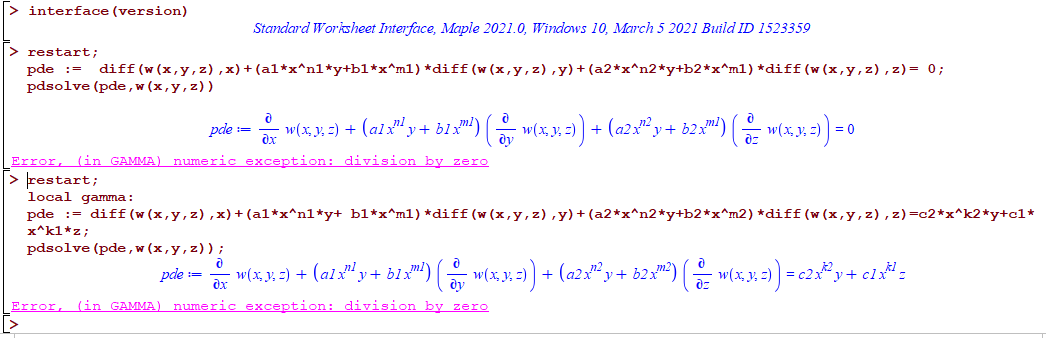Maple 2020.2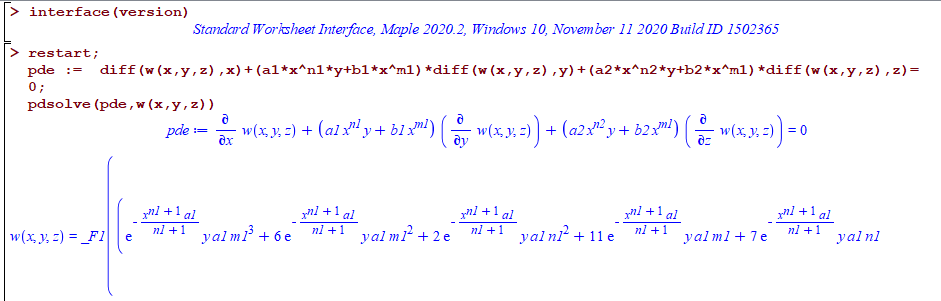All on windows 10.

Do other see the same error? What causes it?

## seq - numelems and declaring a local variable in ...## pdsolve exception int/gbinthm/structure INVALID s...

I noticed number of pde's now fail in Maple 2021 with the error

int/gbinthm/structure INVALID subscript selector

but they do not fail in Maple 2020.2.

Here are few   examples

```restart;
pde :=a*x^n*diff(w(x,y),x) + n*x^m*y*diff(w(x,y),y) =s*x^p*y^q+d;
pdsolve(pde,w(x,y));

restart;
pde :=a*x^n*diff(w(x,y),x) + n*x^m*y*diff(w(x,y),y)=c*x^k*y^s+d;
pdsolve(pde,w(x,y))

restart;
pde :=a*x^n*diff(w(x,y),x)+b*x^m*y*diff(w(x,y),y) =  (c*x^k*y^s + d)*w(x,y);
pdsolve(pde,w(x,y))

restart;
pde :=  a*diff(w(x,y),x)+ y*diff(w(x,y),y) = b*w(x,y)+ c*x^n*y^m;
pdsolve(pde,w(x,y))

#etc..```

Error, (in int/gbinthm/structure) invalid subscript selector

While in Maple 2020.2 they all work. Screen shot

Maple 2021